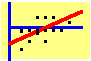8.3 Calculation of the Optimal Portfolio

Elton, Gruber, and Padberg (1976) develop a simple algorithm for calculating optimal portfolios when returns are described by the single-index model. We describe the algorithm first when short sales are permitted, and then move to the case of no short sales.

Recall that in the single-index model, the return from security i is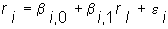The variance of the return is easily calculated to be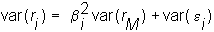The variance consists of two components: the systematic variance, which comes about because of the variance of the index, and the unsystematic or idiosyncratic variance. The portfolio selection problem then reduces to: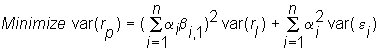subject to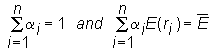Elton, Gruber, and Padberg (1976) start by reformulating the problem. For any portfolio, say P, define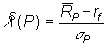This term is called the Sharpe portfolio performance index. It is the excess return (i.e., the return in excess of the risk-free rate) normalized by portfolio risk. This measure is defined for every portfolio with a non-zero variance. In fact, it is also the slope of the line between rf and the portfolio in risk-return space:

Figure 8.1

Construction of Sharpe's Performance

Index for Portfolio P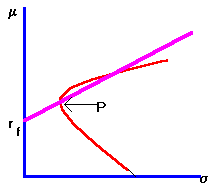If we can find the point with the maximum slope, we will have found the capital market line and also the tangency point (i.e., where the capital markets line touches the minimum-variance frontier). By varying the risk-free interest rate, we can trace out the efficient set. The algorithm finds this tangency point as follows.

Short Sales Permitted

First, compute the "excess return to beta," or Treynor index for every security: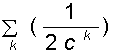(For simplicity, assume that all stocks have positive betas). Compute the value C as follows: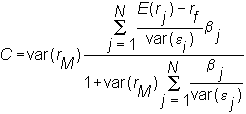Let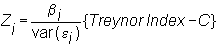The portfolio weights for the tangency point are given by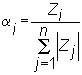You can observe that the portfolio weighting (i.e., long or short) depends upon whether the stock's Treynor index is above or below C.

Short Sales not Permitted

In this case, first rank all securities by their "excess return to beta," or their Treynor index: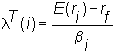(For simplicity, assume that all stocks have positive betas). A simple algorithm identifies the tangency portfolio with no short selling.

Algorithm

Select the stock with the highest Treynor index and compute C for a portfolio consisting of this stock only (i.e., i = 1)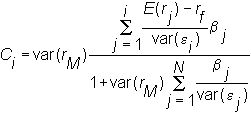If the Treynor index for this stock is greater than Ci, and the index for all other stocks is less than Ci, then the optimal portfolio is attained.

Otherwise repeat the process for a portfolio containing the top two stocks (i.e., i = 2) and so on, and compute a new cutoff value C. The procedure stops when the Treynor index of the last stock added is greater than the cutoff value while the index of the remaining stocks is less than the cutoff value. The optimal portfolio does not include any of the remaining stocks, and the shares of the included stocks are computed in the same way as in the case of short selling.

Multi-Index Models are a natural generalization of the single-index model.

previous topic

next topic